# NY Regents - Circular Arcs and Circles: Help and Review Chapter Exam

Exam Instructions:

Choose your answers to the questions and click 'Next' to see the next set of questions. You can skip questions if you would like and come back to them later with the yellow "Go To First Skipped Question" button. When you have completed the practice exam, a green submit button will appear. Click it to see your results. Good luck!

### Page 1

#### Question 2 2. If angle ACB is 47 degrees, what is the measure of angle AOB?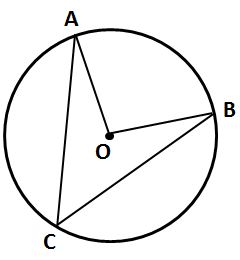#### Question 5 5. In the picture below, if AB is 7, BC is 19 and AD is 8, what is the length of DE?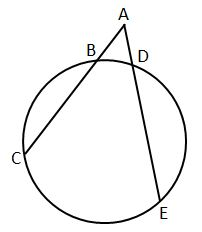### Page 2

#### Question 6 6. In the picture below, if arc AC is 146 degrees and arc BD is 32 degrees, what is the measure of angle AEC?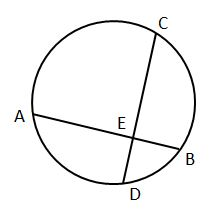#### Question 9 9. If angle AOB is 40 degrees, what is the measure of angle ACB?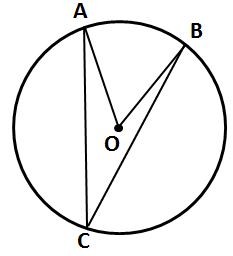#### Question 10 10. If AB and CB are tangent segments, what is the length of CB?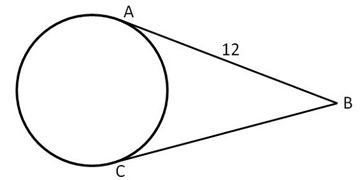### Page 3

#### Question 13 13. Which line is a tangent to the pictured circle?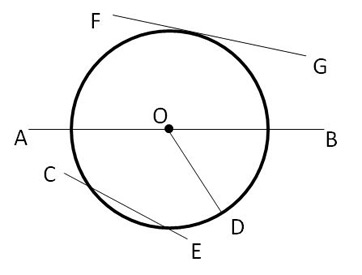#### Question 15 15. In the picture below, if arc BD is 168 degrees and arc BC is 70 degrees, what is the measure of angle BAD?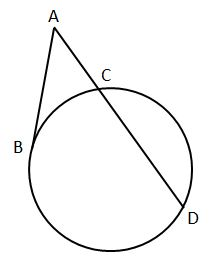### Page 4

#### Question 18 18. If arc AC is 122 degrees and arc BC is 192 degrees, what is the measure of angle ACB?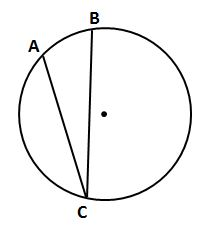#### Question 19 19. In the picture below, if AB is 12, AC is 24 and AD is 10, what is the length of AE?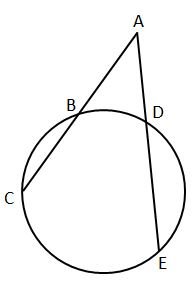#### Question 20 20. In the picture below, if major arc BC is 258 degrees and minor arc BC is 102 degrees, what is the measure of angle BAC?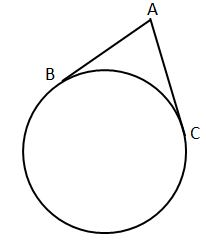### Page 5

#### Question 21 21. If arc BC is 84 degrees, what is the measure of angle ACB?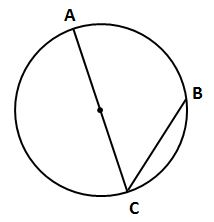#### Question 22 22. If arc AB is 72 degrees, what is the measure of angle ACB?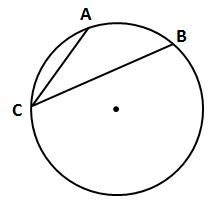#### Question 23 23. If arc AC is 106 degrees and arc BC is 82 degrees, what is the measure of angle ACB?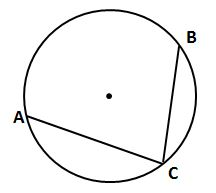#### Question 24 24. If angle ACB is 34 degrees, what is the measure of angle ADB?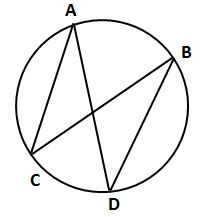### Page 6

#### Question 26 26. In the picture below, if arc CE is 172 degrees and arc BD is 80 degrees, what is the measure of angle CAE?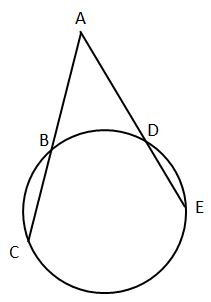#### Question 28 28. In the picture below, if AC is 14 and AB is 10, what is the length of AD?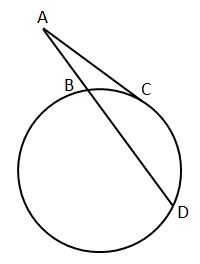#### Question 30 30. If the area of the square is 64, what is the area of the circle, rounded to the nearest whole number?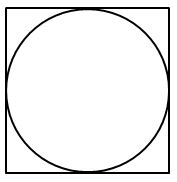#### NY Regents - Circular Arcs and Circles: Help and Review Chapter Exam Instructions

Choose your answers to the questions and click 'Next' to see the next set of questions. You can skip questions if you would like and come back to them later with the yellow "Go To First Skipped Question" button. When you have completed the practice exam, a green submit button will appear. Click it to see your results. Good luck!

Support